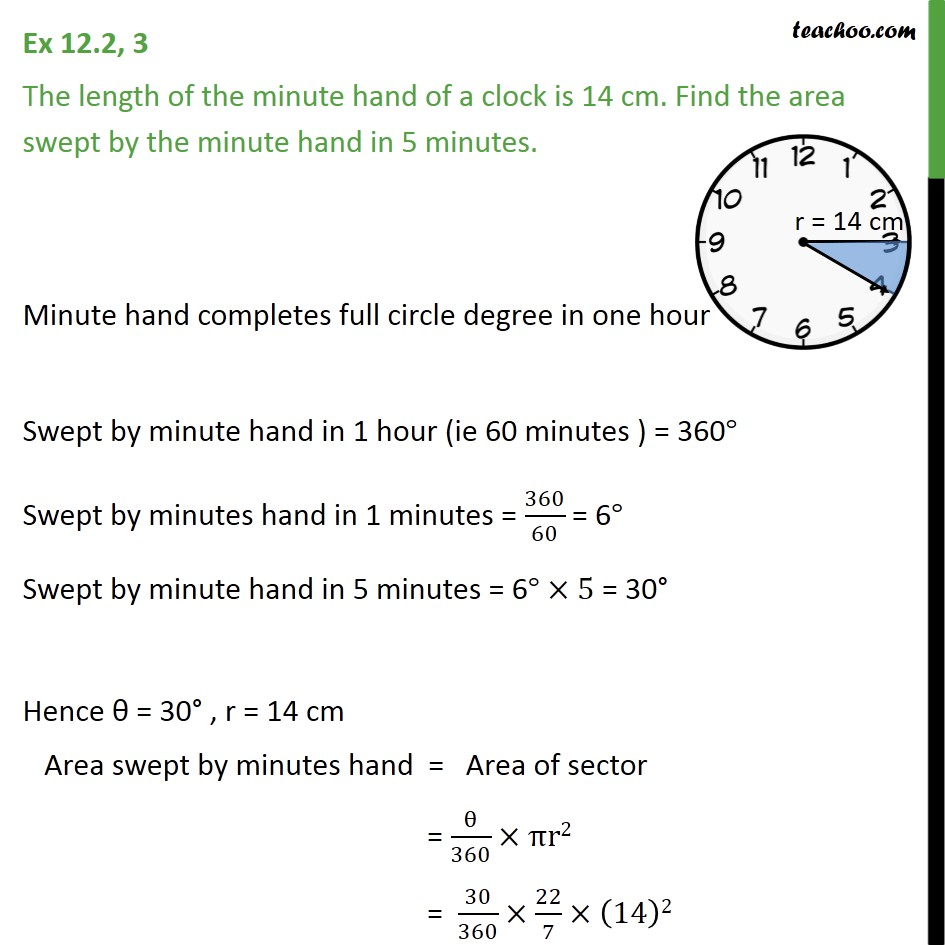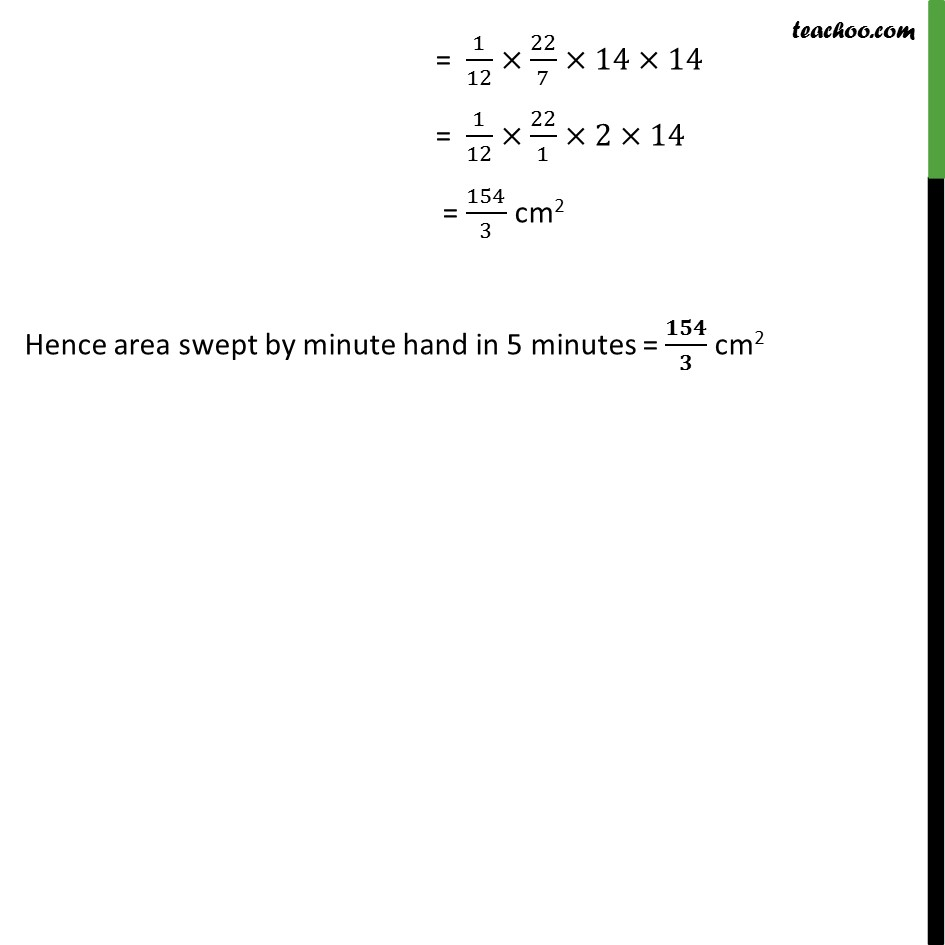1. Chapter 12 Class 10 Areas related to Circles (Term 1)
2. Serial order wise
3. Ex 12.2

Transcript

Ex 12.2, 3 The length of the minute hand of a clock is 14 cm. Find the area swept by the minute hand in 5 minutes. Minute hand completes full circle degree in one hour Swept by minute hand in 1 hour (ie 60 minutes ) = 360° Swept by minutes hand in 1 minutes = 360/60 = 6° Swept by minute hand in 5 minutes = 6° ×5 = 30° Hence θ = 30° , r = 14 cm Area swept by minutes hand = Area of sector = θ/360×πr2 = 30/360×22/7×(14)2 = 1/12×22/7×14×14 = 1/12×22/1×2×14 = 154 cm2 Hence area swept by minute hand in 5 minutes = 154 cm2